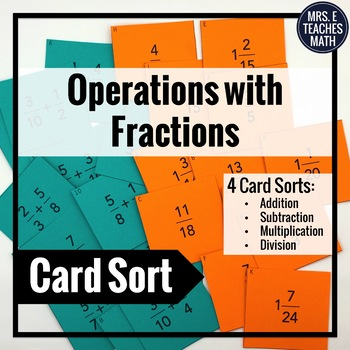# Adding, Subtracting, Multiplying, and Dividing Fractions Activity4th - 6th
Subjects
Standards
Resource Type
Formats Included
• PDF

#### Also included in

1. These NO-PREP activities are going to make your fractions and decimals lessons a hit! Students will love the INTERACTIVE activities and you will love the ease of implementation!This bundle contains the following 15 activities to use throughout a unit on fractions and decimals. Included Activities:A
\$27.48
\$39.75
Save \$12.27

### Description

These READY TO PRINT cards are the perfect HANDS ON practice for your students! You will love that there are FOUR SETS of cards for centers, classroom practice, and more!

In these fractions card sorts, students will match the problem to its solution. They will have to add, subtract, multiply, and divide fractions to find the match.

There are four sets of cards. Each set of cards uses a different operation.

Set 2: Subtracting Fractions

Set 3: Multiplying Fractions

Set 4: Dividing Fractions

These would be great to use in math centers or as individual practice. Each set has 15 cards. Solutions are provided. Download the preview to see problem examples.

~~~~~~~~~~~~~~~~~~~~~~~~~~~~~~~~~~~~~~~~~~~~~~~~~~~~~~~~~

This is also part of the following bundle:

Fractions and Decimals Activity Bundle

You may be interested in my other Fractions and Decimals products:

Fraction, Decimal, Percent Cut-Out Puzzle

Mixed Numbers Word Problems Stations Maze: Adding and Subtracting

Multiplying and Dividing Fractions Which is Largest Activity

Adding and Subtracting Decimals Which is Largest Activity

Adding and Subtracting Fractions Which is Largest Activity

Multiplying and Dividing Decimals Which is Largest Activity

Multiplying Decimals Word Problems Stations Maze

Multiplying and Dividing Decimals Ladder Activity

Mixed Numbers and Improper Fractions Square Puzzle

Operations with Decimals Color by Number

~~~~~~~~~~~~~~~~~~~~~~~~~~~~~~~~~~~~~~~~~~~~~~~~~~~~~~~~~

If you have any questions or comments please email me at: mrseteachesmath@gmail.com.

This purchase is for one teacher only.

Purchasing this product grants permission for use by one teacher in his or her own classroom. This item is bound by copyright laws and redistributing, editing, selling, or posting this item (or any part thereof) on the Internet are all strictly forbidden. If you wish to share with colleagues, please purchase additional licenses.

Thank you!

Total Pages
Included
Teaching Duration
N/A
Report this Resource to TpT
Reported resources will be reviewed by our team. Report this resource to let us know if this resource violates TpT’s content guidelines.

### Standards

to see state-specific standards (only available in the US).
Apply and extend previous understandings of division to divide unit fractions by whole numbers and whole numbers by unit fractions.
Explaining why multiplying a given number by a fraction greater than 1 results in a product greater than the given number (recognizing multiplication by whole numbers greater than 1 as a familiar case); explaining why multiplying a given number by a fraction less than 1 results in a product smaller than the given number; and relating the principle of fraction equivalence 𝘢/𝘣 = (𝘯×𝘢)/(𝘯×𝘣) to the effect of multiplying 𝘢/𝘣 by 1.
Add and subtract fractions with unlike denominators (including mixed numbers) by replacing given fractions with equivalent fractions in such a way as to produce an equivalent sum or difference of fractions with like denominators. For example, 2/3 + 5/4 = 8/12 + 15/12 = 23/12. (In general, 𝘢/𝘣 + 𝘤/𝘥 = (𝘢𝘥 + 𝘣𝘤)/𝘣𝘥.)